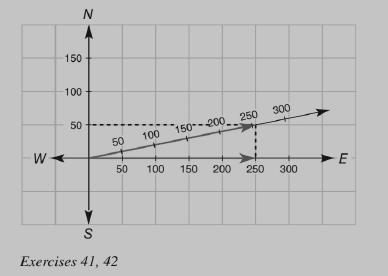Chapter 4.1, Problem 39E### Elementary Geometry for College St...

6th Edition
Daniel C. Alexander + 1 other
ISBN: 9781285195698

#### Solutions

Chapter
Section### Elementary Geometry for College St...

6th Edition
Daniel C. Alexander + 1 other
ISBN: 9781285195698
Textbook Problem
1 views

# The following problem is based on the Parallelogram Law. In the scaled drawing, each unit corresponds to 50 mph. A small airplane travels due east at 250 mph. The wind is blowing at 50 mph in the direction due north. Using the indicated diagonal and use it to determine the speed of the airplane in miles per hour.To determine

The speed of the airplane in miles per hour using the indicated diagonal.

Explanation

Given:

In the scaled drawing given below, each unit corresponds to 50 mph. A small airplane travels due east at 250 mph. The wind is blowing at 50 mph in the direction due north.

Parallelogram Law:

Enables to determine the speed and direction of an airplane when the velocity of the airplane and that of the wind are considered together...

### Still sussing out bartleby?

Check out a sample textbook solution.

See a sample solution

#### The Solution to Your Study Problems

Bartleby provides explanations to thousands of textbook problems written by our experts, many with advanced degrees!

Get Started

#### Find more solutions based on key concepts